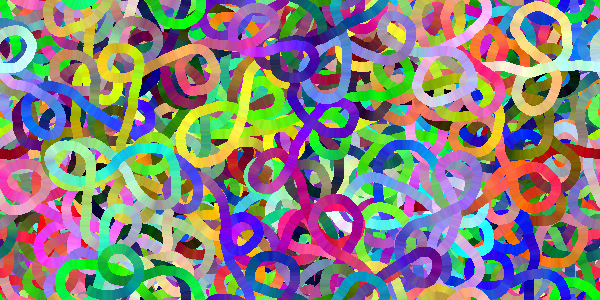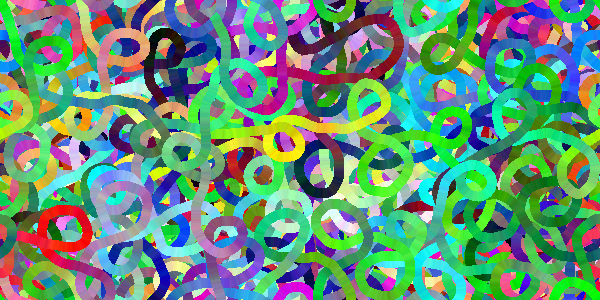# Worms

example processing animation generative-art

This code uses a heading (an angle to slither towards), the `sin()` function (which generates values that alternate from `-1` to `1` to move the heading left and right), and the `randomGaussian()` function (which generates random numbers centered around `0` to add some randomness to the movement) to create a point that loops around like a worm.

The code also uses the `cos()` and `sin()` functions to figure out the left and right sides of the worm based on the point and the heading. Then it fills in the space between the previous left and right points and the current left and right points to draw the worm.

And just for fun, that space is colored with a randomly changing value to make it a rainbow worm. 🌈🐛

``````float heading;
float x;
float y;
float speed = 1;
float thickness = 5;

float r = 128;
float g = 128;
float b = 128;

float prevLeftX;
float prevLeftY;
float prevRightX;
float prevRightY;

void setup() {
size(500, 500);
noSmooth();
frameRate(500);
background(64);
}

void draw() {

r += random(-10, 10);
g += random(-10, 10);
b = constrain(b, 0, 256);

r = constrain(r, 0, 256);
g = constrain(g, 0, 256);
b += random(-10, 10);

if (x < 0) {
x = width;
prevLeftX += width;
prevRightX += width;
}
if (x > width) {
x = 0;
prevLeftX -= width;
prevRightX -= width;
}

if (y < 0) {
y = height;
prevLeftY += height;
prevRightY += height;
}
if (y > height) {
y = 0;
prevLeftY -= height;
prevRightY -= height;
}

noStroke();
fill(r, g, b);

beginShape();
vertex(prevLeftX, prevLeftY);
vertex(leftX, leftY);
vertex(rightX, rightY);
vertex(prevRightX, prevRightY);
endShape(CLOSE);

prevLeftX = leftX;
prevLeftY = leftY;
prevRightX = rightX;
prevRightY = rightY;
}
``````
Code Editor

See the Pen by Happy Coding (@KevinWorkman) on CodePen.

If this seems complicated, try modifying the code. Take this line:

``````heading += sin(radians(frameCount))*3 + randomGaussian()*4;
``````

This line uses both the `sin()` function to generate the winding left and right motion and the `randomGaussian()` to add a bit of randomness to that. Try only using the `sin()` function or the `randomGaussian()` to see how the worm moves.🐛🐛🐛## Tweak Ideas

• Modify the `heading +=` line to change how the worm moves.
• Make the worm look more realistic, or like a snake. 🐍